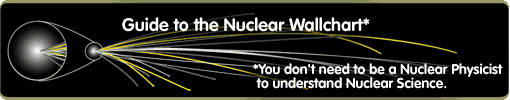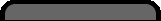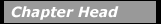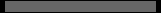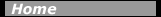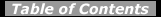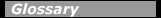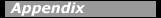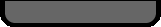## Beta Decay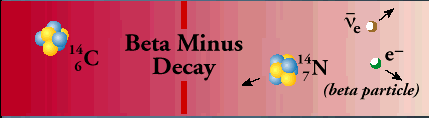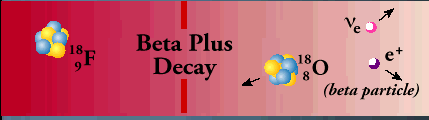Beta decays

Beta particles are electrons or positrons (electrons with positive electric charge, or antielectrons). Beta decay occurs when, in a nucleus with too many protons or too many neutrons, one of the protons or neutrons is transformed into the other. In beta minus decay, a neutron decays into a proton, an electron, and an antineutrino: n Æ p + e - +. In beta plus decay, a proton decays into a neutron, a positron, and a neutrino: p Æ n + e+ +n. Both reactions occur because in different regions of the Chart of the Nuclides, one or the other will move the product closer to the region of stability. These particular reactions take place because conservation laws are obeyed. Electric charge conservation requires that if an electrically neutral neutron becomes a positively charged proton, an electrically negative particle (in this case, an electron) must also be produced. Similarly, conservation of lepton number requires that if a neutron (lepton number = 0) decays into a proton (lepton number = 0) and an electron (lepton number = 1), a particle with a lepton number of -1 (in this case an antineutrino) must also be produced. The leptons emitted in beta decay did not exist in the nucleus before the decay–they are created at the instant of the decay.

To the best of our knowledge, an isolated proton, a hydrogen nucleus with or without an electron, does not decay. However within a nucleus, the beta decay process can change a proton to a neutron. An isolated neutron is unstable and will decay with a half-life of 10.5 minutes. A neutron in a nucleus will decay if a more stable nucleus results; the half-life of the decay depends on the isotope. If it leads to a more stable nucleus, a proton in a nucleus may capture an electron from the atom (electron capture), and change into a neutron and a neutrino.

Proton decay, neutron decay, and electron capture are three ways in which protons can be changed into neutrons or vice-versa; in each decay there is a change in the atomic number, so that the parent and daughter atoms are different elements. In all three processes, the number A of nucleons remains the same, while both proton number, Z, and neutron number, N, increase or decrease by 1.

In beta decay the change in binding energy appears as the mass energy and kinetic energy of the beta particle, the energy of the neutrino, and the kinetic energy of the recoiling daughter nucleus. The energy of an emitted beta particle from a particular decay can take on a range of values because the energy can be shared in many ways among the three particles while still obeying energy and momentum conservation.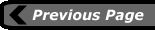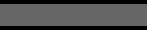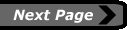last updated: August 9, 2000 webmaster